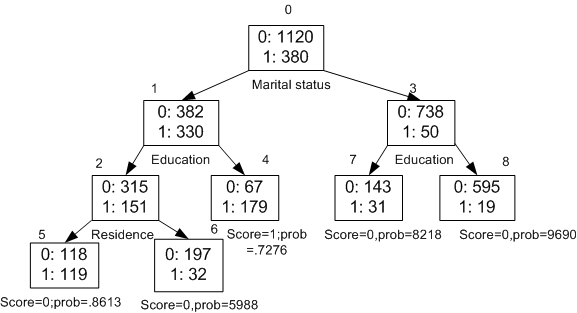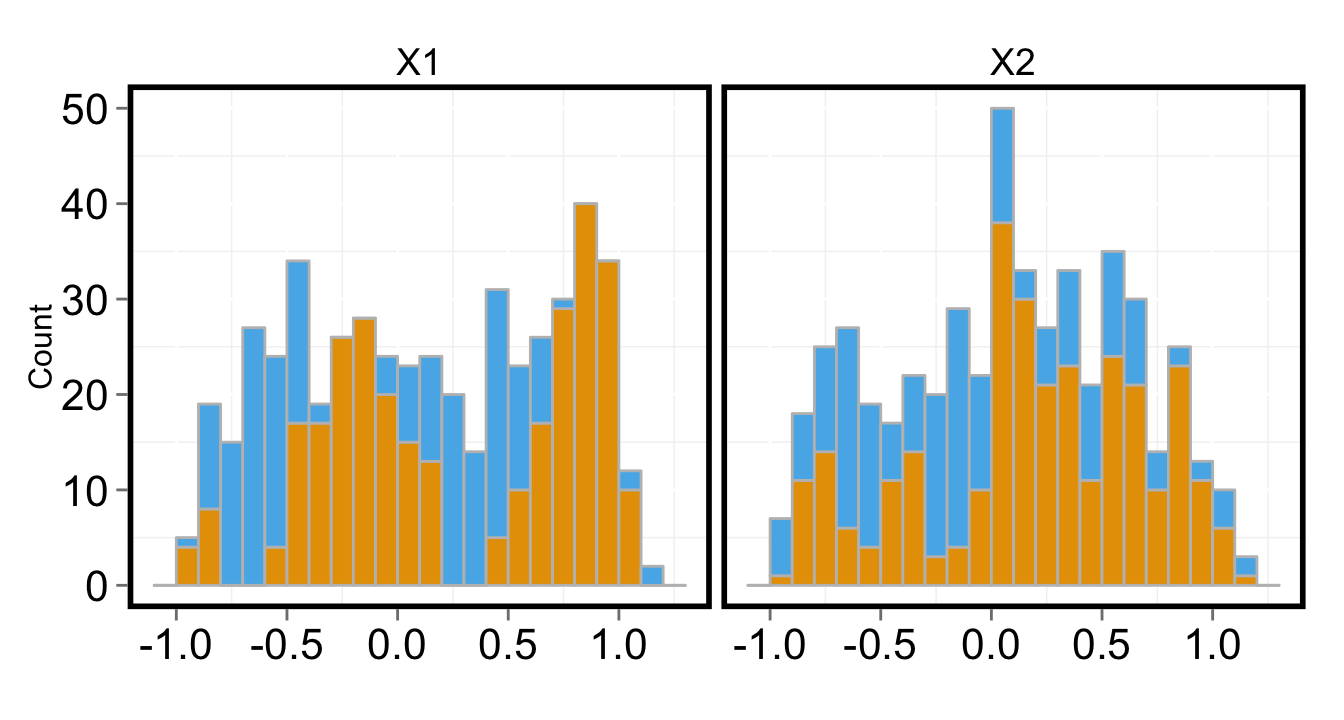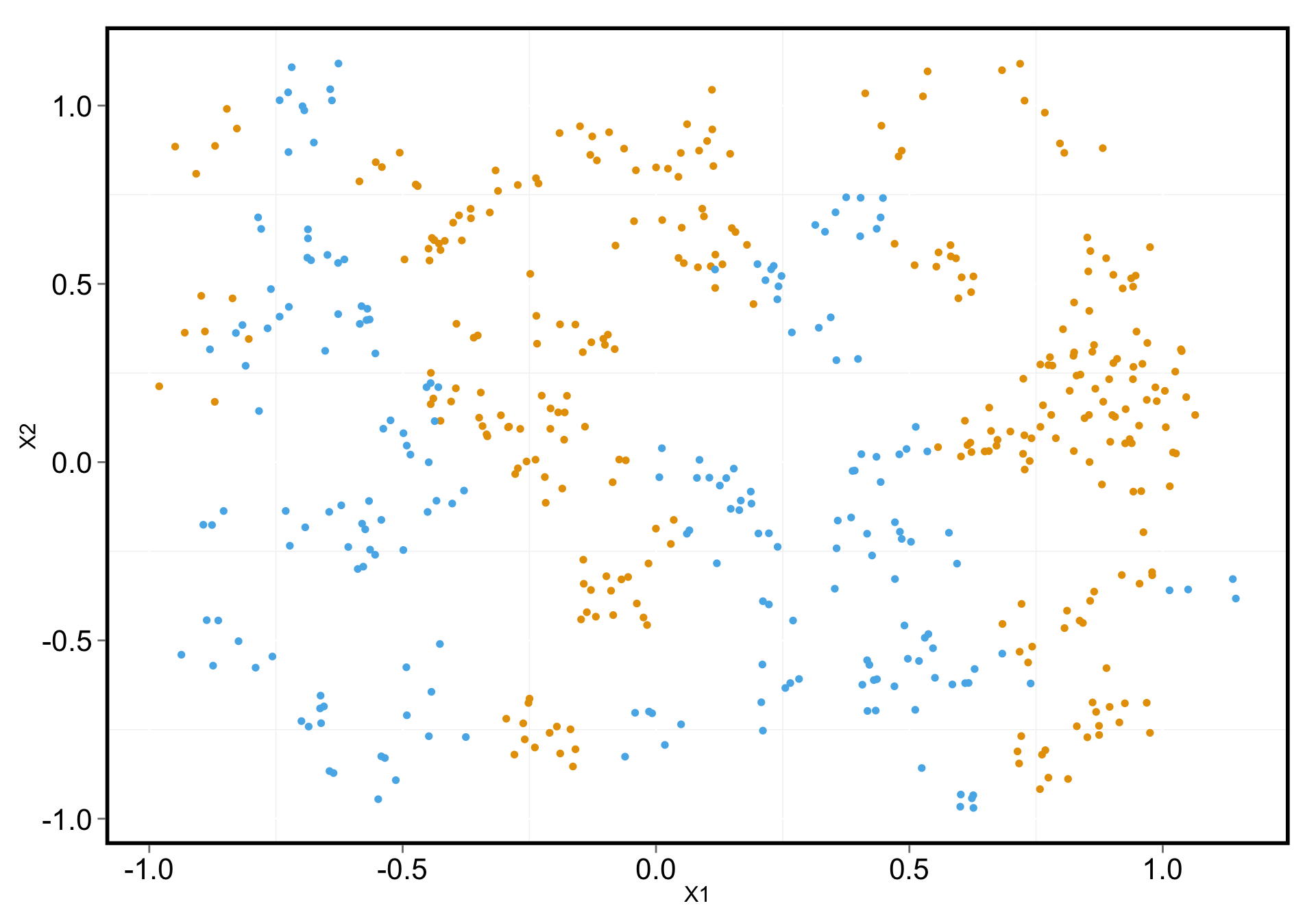April 9, 2015

# Classification trees

Classification trees are a method commonly used in data mining. They are popular becase they are easy to interpret and reflect how (some) decisions are made.# Classification trees

The basic idea is to (recursively) divide the feature space into regions and fit a simple ‘model’ for each region.

Details:

• Grow in top-down fasion (divisive) by choosing the split that optimizies the criterion (greedy).
• Grow a large tree and then prune it at the end.

We can estimate the probability that the class label is $$k$$ given that the feature vector lies in region $$M$$.

# Some things to consider:

How do we choose the best splits?

We want splits that best separate our class labels.

How large do we grow the tree (when do we stop splitting?)

We want the number of observations in the terminal node to be reasonably large otherwise we are just modeling noise.

Stopping rules:

1. If all observations belong to the same class.
2. Too few observations to continue splitting.

# Split criterions

There are several different methods for choosing the best split.

• Misclassification error
• Gini
• Cross-entropy (deviance)

# Misclassification error

The proportion of the observations in that region that do not belong to the most common class.

$\text{Error} = 1 - \text{max}(\hat{p}_{mk})$

# Gini

Measures the total variance across the $$K$$ classes.

Small values indicates that a node contains predominantly observations from a single class (i.e. small variation).

Attempts to separate classes by focusing on one class at a time. It will always favor working on the largest or, if you use costs or weights, the most “important” class in a node.

$\text{Gini} = \overset{K}{\underset{k = 1}{\Sigma}} \hat{p}_{mk} (1 - \hat{p}_{mk})$

# Cross-entropy (deviance)

Similar measure of node purity where small values also indicate node purity (like Gini).

Instead of separating one class at a time entropy tends to separate groups of classes, each accounting for roughly 50% of the observations.

Results in more evenly balanced trees.

$\text{Deviance} = -\overset{K}{\underset{k = 1}{\Sigma}} \hat{p}_{mk} \text{log} \hat{p}_{mk}$

# So which split criterion should you use?

It doesn’t really matter. Different split criterions usually give similar performance.

The tree package uses defaults to deviance; random forests uses Gini.# Two-dimensional example

# Summary of dataset
summary(train_data)
##     Group           X1                X2
##  Orange:297   Min.   :-0.9804   Min.   :-0.96966
##  Blue  :203   1st Qu.:-0.3941   1st Qu.:-0.34460
##               Median : 0.1148   Median : 0.09573
##               Mean   : 0.1261   Mean   : 0.07507
##               3rd Qu.: 0.6584   3rd Qu.: 0.52230
##               Max.   : 1.1442   Max.   : 1.11792# Two-dimensional example

# Print random 5 rows
head(train_data[sample(nrow(train_data)),])
##      Group          X1         X2
## 110   Blue  0.35868128 -0.1638366
## 45  Orange -0.34901851  0.1246819
## 66  Orange  0.90250625  0.5254130
## 438 Orange  0.05070714  0.6575863
## 324 Orange  0.76363161  0.1595582
## 14  Orange  0.81636073  0.2001557

# Fitting the tree

# Load the tree package
library(tree)
# Fit the classification tree
tree_fit <- tree(Group ~ X1 + X2, data = train_data)
# Model summary
summary(tree_fit)
##
## Classification tree:
## tree(formula = Group ~ X1 + X2, data = train_data)
## Number of terminal nodes:  17
## Residual mean deviance:  0.242 = 116.9 / 483
## Misclassification error rate: 0.042 = 21 / 500
# Plot the dendrogram
plot(tree_fit, type = 'uniform')
text(tree_fit)

# Dendrogram

# Plot the dendrogram
plot(tree_fit, type = 'uniform')
text(tree_fit, all = TRUE)